Kruskal-Katona theorem

(Redirected from Kruskal–Katona theorem)

Letand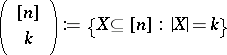,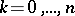(cf. also Sperner property; Sperner theorem). The elements of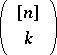can be linearly ordered in the following way: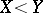if the largest element in whichanddiffer is in(cf. also Totally ordered set). If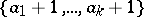,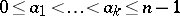, is the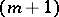th element in this order, then(a1)

since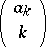-element subsets ofhave maximal element smaller than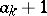,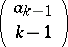have maximal elementbut the second largest element smaller than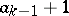, etc.. Equation (a1) is called the-representation of,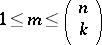(for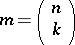, one takes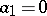,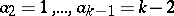,). This (unique) representation can be found directly by choosing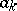maximal such that,maximal such that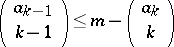, etc..

For a family, the lower shadow ofis defined by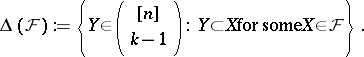Ifis given as above, then the lower shadow of the family of the firstelements inis the family of all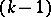-element subsets of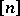having maximal element smaller than, of all-element subsets ofhaving maximal element, but the second largest element smaller than, etc., i.e. the family of the firstelements of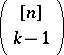whereThe Kruskal–Katona theorem states that in this way one obtains a lower shadow of minimum size, i.e., if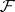is any-element family inandis given by (a1), thenThere exist generalizations to similar results for other partially ordered sets, like products of chains, products of stars, the partially ordered set of subwords of--words, and the partially ordered set of submatrices of a matrix.

The following result of L. Lovász is weaker but numerically easier to handle: If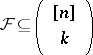and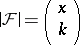with some real, where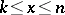, then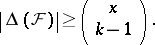The original papers by J.B. Kruskal and G.O.H. Katona are [a3], [a4].

According to [a2], p. 1296, the Kruskal–Katona theorem is probably the most important one in finite extremal set theory.